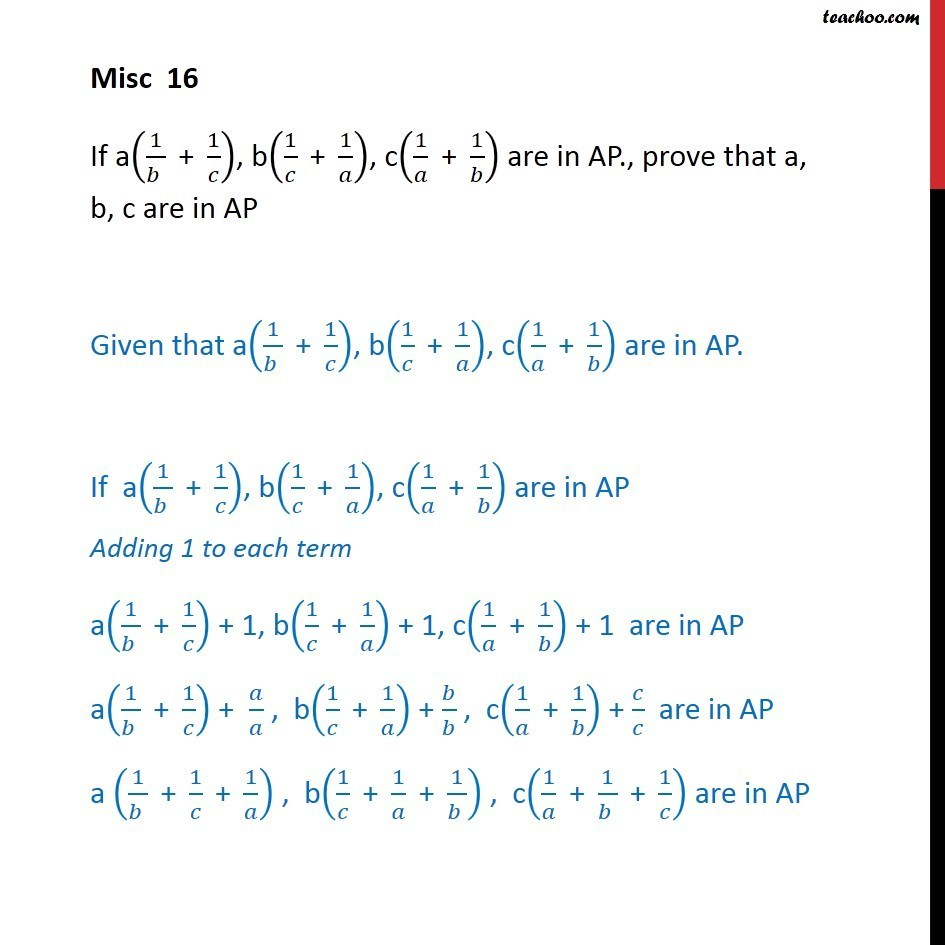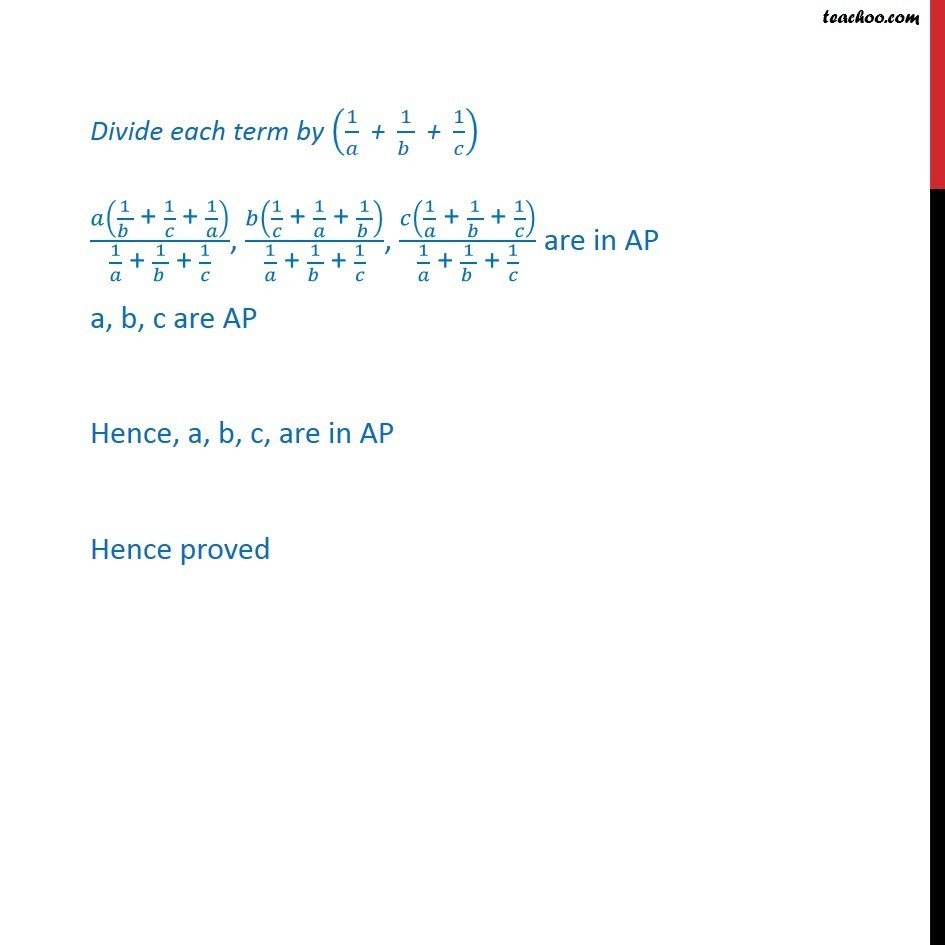Miscellaneous

Chapter 8 Class 11 Sequences and Series
Serial order wiseLearn in your speed, with individual attention - Teachoo Maths 1-on-1 Class

### Transcript

Misc 16 If a(1/( ) " + " 1/ ), b(1/ " + " 1/ ), c(1/ " + " 1/ ) are in AP., prove that a, b, c are in AP Given that a(1/( ) " + " 1/ ), b(1/ " + " 1/ ), c(1/ " + " 1/ ) are in AP. If a(1/( ) " + " 1/ ), b(1/ " + " 1/ ), c(1/ " + " 1/ ) are in AP Adding 1 to each term a(1/( ) " + " 1/ ) + 1, b(1/ " + " 1/ ) + 1, c(1/ " + " 1/ ) + 1 are in AP a(1/( ) " + " 1/ ) + / , b(1/ " + " 1/ ) + / , c(1/ " + " 1/ ) + / are in AP a (1/( ) " + " 1/ " + " 1/ ) , b(1/ " + " 1/ " + " 1/( )) , c(1/ " + " 1/( ) " + " 1/ ) are in AP Divide each term by (1/ " + " 1/( ) " + " 1/ ) (1/( ) " + " 1/ " + " 1/ )/(1/ " + " 1/( ) " + " 1/ ), (1/ " + " 1/ " + " 1/( ))/(1/ " + " 1/( ) " + " 1/ ), (1/ " + " 1/( ) " + " 1/ )/(1/ " + " 1/( ) " + " 1/ ) are in AP a, b, c are AP Hence, a, b, c, are in AP Hence proved